# Group (mathematics)

In mathematics, a group is a set and an operation that combines any two elements of the set to produce a third element of the set, in such a way that the operation is associative, an identity element exists and every element has an inverse. These three axioms hold for number systems and many other mathematical structures. For example, the integers together with the addition operation form a group. The concept of a group and the axioms that define it were elaborated for handling, in a unified way, essential structural properties of very different mathematical entities such as numbers, geometric shapes and polynomial roots. Because the concept of groups is ubiquitous in numerous areas both within and outside mathematics, some authors consider it as a central organizing principle of contemporary mathematics. In geometry groups arise naturally in the study of symmetries and geometric transformations: The symmetries of an object form a group, called the symmetry group of the object, and the transformations of a given type form a general group. Lie groups appear in symmetry groups in geometry, and also in the Standard Model of particle physics. The Poincaré group is a Lie group consisting of the symmetries of spacetime in special relativity. Point groups describe symmetry in molecular chemistry. The concept of a group arose in the study of polynomial equations, starting with Évariste Galois in the 1830s, who introduced the term group (French: groupe) for the symmetry group of the roots of an equation, now called a Galois group. After contributions from other fields such as number theory and geometry, the group notion was generalized and firmly established around 1870. Modern group theory—an active mathematical discipline—studies groups in their own right. To explore groups, mathematicians have devised various notions to break groups into smaller, better-understandable pieces, such as subgroups, quotient groups and simple groups. In addition to their abstract properties, group theorists also study the different ways in which a group can be expressed concretely, both from a point of view of representation theory (that is, through the representations of the group) and of computational group theory. A theory has been developed for finite groups, which culminated with the classification of finite simple groups, completed in 2004. Since the mid-1980s, geometric group theory, which studies finitely generated groups as geometric objects, has become an active area in group theory. (Wikipedia).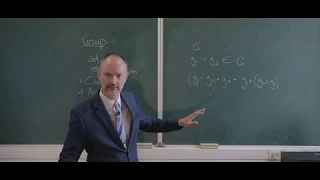Definition of a group Lesson 24

In this video we take our first look at the definition of a group. It is basically a set of elements and the operation defined on them. If this set of elements and the operation defined on them obey the properties of closure and associativity, and if one of the elements is the identity el

From playlist Abstract algebra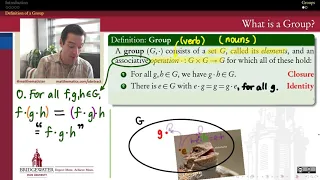301.2 Definition of a Group

A group is (in a sense) the simplest structure in which we can do the familiar tasks associated with "algebra." First, in this video, we review the definition of a group.

From playlist Modern Algebra - Chapter 15 (groups)What is a Group? | Abstract Algebra

Welcome to group theory! In today's lesson we'll be going over the definition of a group. We'll see the four group axioms in action with some examples, and some non-examples as well which violate the axioms and are thus not groups. In a fundamental way, groups are structures built from s

From playlist Abstract Algebra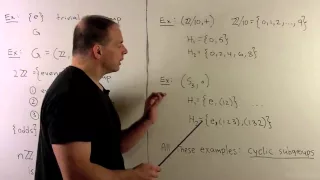GT2. Definition of Subgroup

Abstract Algebra: We define the notion of a subgroup and provide various examples. We also consider cyclic subgroups and subgroups generated by subsets in a given group G. Example include A4 and D8. U.Reddit course materials available at http://ureddit.com/class/23794/intro-to-group-

From playlist Abstract Algebra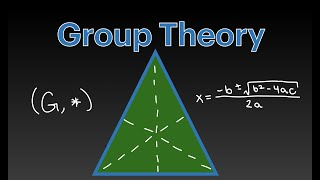What is Group Theory?

This video contains the origins of group theory, the formal definition, and theoretical and real-world examples for those beginning in group theory or wanting a refresher :)

From playlist Summer of Math Exposition Youtube Videos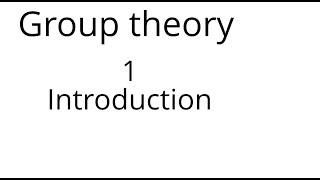Group theory 1: Introduction

This is lecture 1 of an online mathematics course on group theory. This lecture defines groups and gives a few examples of them.

From playlist Group theory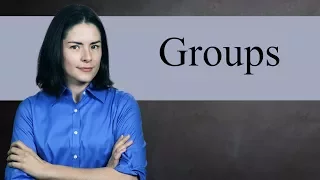Group Definition (expanded) - Abstract Algebra

The group is the most fundamental object you will study in abstract algebra. Groups generalize a wide variety of mathematical sets: the integers, symmetries of shapes, modular arithmetic, NxM matrices, and much more. After learning about groups in detail, you will then be ready to contin

From playlist Abstract Algebra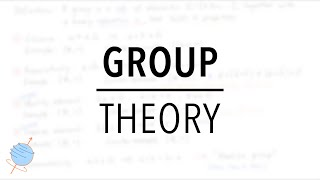Group Theory for Physicists (Definitions with Examples)

In this video, we cover the most basic points that a physicist should know about group theory. Along the way, we'll give you lots of examples that illustrate each step. 00:00 Introduction 00:11 Definition of a Group 00:59 (1) Closure 01:34 (2) Associativity 02:02 (3) Identity Element 03:

From playlist Mathematical Physics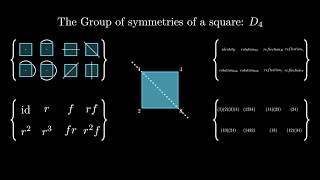What is a Group (Intuitively) - Group Theory 001

Here we begin to explore the mathematical objects that describe symmetry - Groups, and look at one particular example: the group of symmetries of a square 0:00 - Intro 0:25 - What is a group? 0:49 - Simple mathematical object example 1:49 - Symmetries 3:09 - A group is 3:20 - The group of

From playlist Summer of Math Exposition Youtube Videos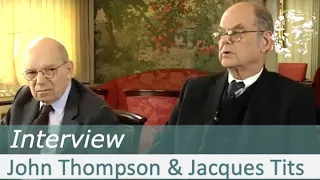John Thompson and Jacques Tits - The Abel Prize interview 2008

0:21 Interest in and contributions to mathematics in childhood / early youth 3:56 Group theory and Niels Henrik Abel 5:46 Why simple groups are important for classification for finite groups in general - Decomposition theorem 6:52 Group theory: Peter Ludwig Mejdell Sylow and Sophus Lie 7:2

From playlist John Griggs Thompson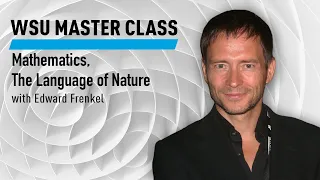WSU Master Class: Mathematics, The Language of Nature with Edward Frenkel Course

Join mathematician Edward Frenkel as he discusses how the elegant mathematical formulation of symmetry has been used throughout math and physics and could, through the Langlands program, give rise to a grand unified theory of mathematics. This lecture was recorded on May 31, 2014, at the

From playlist WSU Master ClassesWSU Master Class: Mathematics, The Language of Nature with Edward Frenkel Course

Join mathematician Edward Frenkel as he discusses how the elegant mathematical formulation of symmetry has been used throughout math and physics and could, through the Langlands program, give rise to a grand unified theory of mathematics. This lecture was recorded on May 31, 2014, at the

From playlist WSU Master Class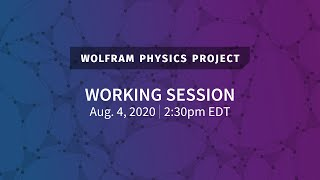Wolfram Physics Project: Working Session Tuesday, Aug. 4, 2020 [Empirical Physical Metamathematics]

This is a Wolfram Physics Project working session on empirical physical metamathematics. Stephen discusses this in A New Kind of Science: https://www.wolframscience.com/nks/notes-12-9--empirical-metamathematics/ Originally livestreamed at: https://twitch.tv/stephen_wolfram Stay up-to-dat

From playlist Wolfram Physics Project Livestream Archive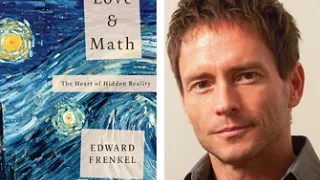Elementary Introduction to the Langlands Program, by Edward Frenkel (Part 1) 

"Do we discover mathematics or do we invent it?" One of the most fascinating and important developments in mathematics in the last 50 years is the Langlands Program, a collection of ideas that provides a grand unification of many areas of mathematics. In September 2015, Edward Frenkel g

From playlist Number Theory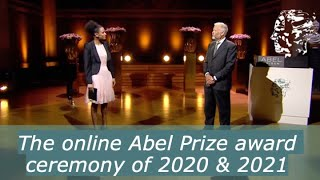Abel Prize award ceremony 2021

The ceremony honours both the 2020-winners, Hillel Furstenberg and Gregory Margulis, and the 2021-winners, Avi Wigderson and László́ Lovász. 0:30 Haddy N'jie sings Feeling Good 3:18 Welcome by Master of ceremonies, Haddy N'jie 4:46 On the nomination process and the work of the Abel Prize

From playlist Gregory Margulis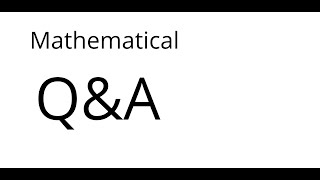This is an experimental video where I give answers to the (mostly) mathematical questions asked by viewers.

From playlist Math talks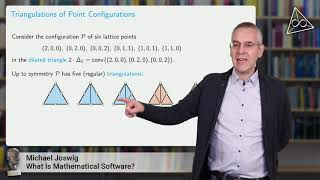Michael Joswig - What is Mathematical Software

What Is Mathematical Software? A short answer to this question is: Mathematical Software is what mathematics receives as a benefit from the digital age. This is relevant because Mathematical Software is useful in many ways. For instance, Mathematical Software serves as a tool to support

From playlist Research Spotlight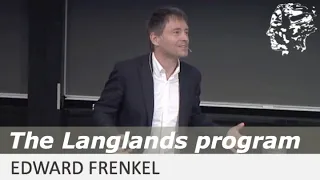Edward Frenkel: Langlands Program and Unification

Abstract: Sophia Kovalevskaya wrote, "It is not possible to be a mathematician without being a poet at heart. A poet should see what others can’t see, see deeper than others. And that’s the job of a mathematician as well.” The work of Robert Langlands sets a great example for this maxim, a

From playlist Abel LecturesGroup Theory: The Center of a Group G is a Subgroup of G Proof

Please Subscribe here, thank you!!! https://goo.gl/JQ8Nys Group Theory: The Center of a Group G is a Subgroup of G Proof

From playlist Abstract Algebra